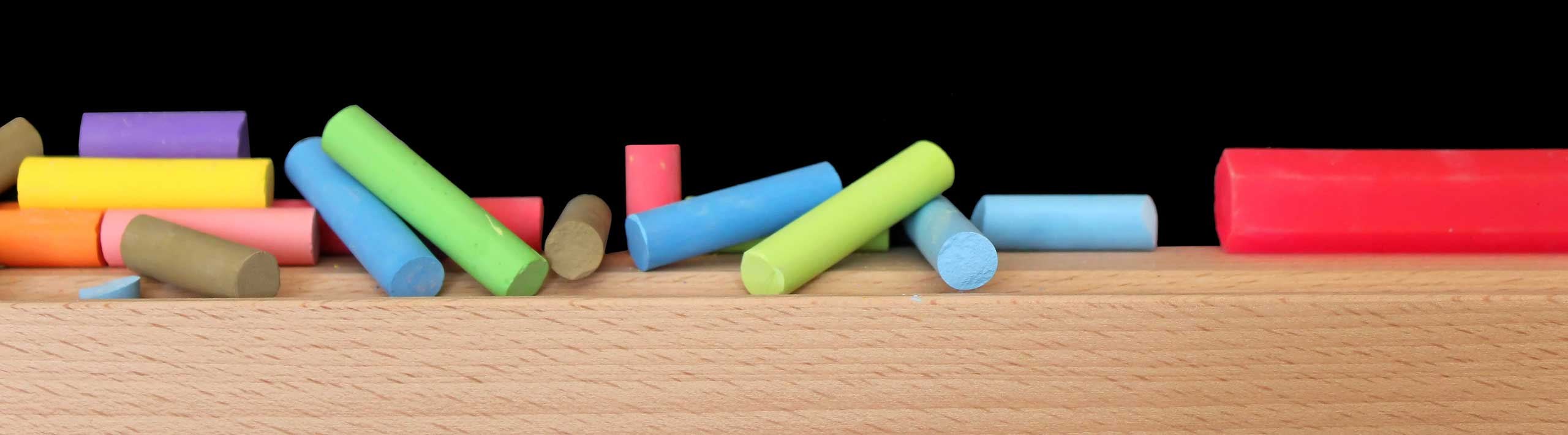# Convert Time from Decimal to Hours, Minutes and Seconds

An online calculator to convert time from decimal to hours, minutes and seconds using the facts that
1 hour = 60 minutes
1 minute = 60 seconds.

## Example

Convert 2.13 hours to hours, minutes and seconds.
The whole part 2 is the number of hours
The decimal part 0.13 is less than one hour and is therefore converted to minutes as follows
0.13 hours = 0.13 � 60 minutes = 7.8 minutes
The whole part 7 in 7.8 is the number of minutes
The decimal part 0.8 minutes is now converted in seconds as follows
0.8 minutes = 0.8 � 60 seconds = 48 seconds.
Hence
2.13 hours = 2 hours , 7 minutes and 48 seconds.

## How to use the calculator

1 - Enter the time in decimal format press "enter". The answer is in hours, minutes and seconds. 2.13 hours, converted analytically above, is the default input to the calculator. Input more examples and check them analytically.

 2.13 hours =